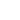Hot Topics

# Formula for Time in Excel

mgmcc 16:09 05 Jul 2009
Locked

I'm looking for the formula to subtract one time from another and give the answer in hours to 2 decimal places, not hours and minutes.

In other words, Start 08:00 Finish 18:15 - I want an answer of 10.25 hours, not 10:15 (ten hours, 15 mins).

Simsy 18:36 05 Jul 2009

of how you format the cell... and how you have the formula...

You need to have the cell with the answer in formatted as a number, (to 2 decimal places, as that's what you seem to want). This will give the answer as a fraction of 1 day...

Then have your formula, (=B2-A2), and multiply it by 24 to give the answer in hours...

eg if the start is in A2, and the end is in B2, the fomula would be;

=(B2-A2)*24

Regards,

Simsy

mgmcc 18:59 05 Jul 2009

Many thanks, exactly what I was looking for.

mgmcc 13:49 06 Jul 2009

Some of the times do span midnight but I'd actually got round this with an "IF" statement - if the subtraction resulted in a negative number, 24 was added to it. However, I'll have a play about with your formula.

Thanks.

This thread is now locked and can not be replied to.Elsewhere on IDG sites

7 ways Alexa can make lockdown more bearable

Facebook Shops: How and when can I sell on Facebook and Instagram?

Different Think: Why Apple needs to stop being so cheap

Confidentialité : comment bloquer les pubs et empêcher les annonceurs de vous suivre ?